Next: Texture Synthesis Up: Parallel Algorithm Analysis Previous: CM-2 Communications Model

## Complexity of Some Basic Operations

A two-dimensional Fast Fourier Transform (FFT) is a commonly used technique in digital image processing, and several algorithms in this paper make use of it. The FFT is well-suited for parallel applications because it is efficient and inherently parallel (, , , , ). With an image size of n elements, O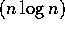operations are needed for an FFT. On a parallel machine with p processors, O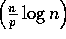computational steps are required. The communications needed for an FFT are determined by the FFT algorithm implemented on a particular parallel machine. The CM-2 pipelines computations using successive butterfly stages . Its total time complexity is given by: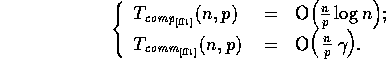On the CM-5, however, this algorithm would not be efficient. Instead, a communications efficient algorithm described in  is used, and has complexity: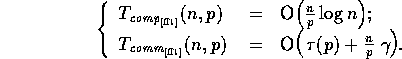for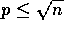.

Another extensively used and highly parallel primitive operation is the Scan operation. Given an array A with n elements,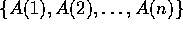, its scan will result in array C, where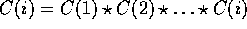and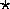is any associative binary operation on the set, such as addition, multiplication, minimum, and maximum, for real numbers. A scan in the forward direction yields the parallel-prefix operation, while a reverse scan is a parallel-suffix. We can extend this operation to segmented scans, where we also input an n element array B of bits, such that for each i,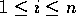, the element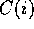equals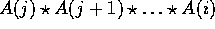, where j is the largest index of segment array B with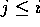and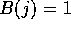.

A scan operation on a sequential machine obviously takes O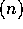operations. An efficient parallel algorithm uses a binary tree to compute the scan in O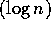time with Ooperations . On the CM-2, the complexity for a scan is given by: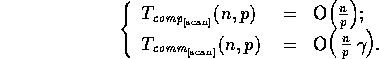The CM-5 efficiently supports scans in hardware  and has complexity: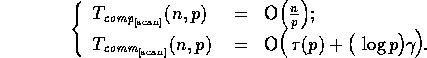As the above complexities show, these algorithms efficiently scale for problem and machine size.

The data parallel programming paradigm is ideally suited for image processing since a typical task consists of updating each pixel value based on the pixel values in a small neighborhood. Assuming the existence of sufficiently many virtual processors, this processing task can be completed in time proportional to the neighborhood size. There are several powerful techniques for developing data parallel algorithms including scan (prefix sums) operations, divide-and-conquer, partitioning (data and function), and pipelining. We use several of these techniques in our implementations of texture synthesis and compression algorithms.Next: Texture Synthesis Up: Parallel Algorithm Analysis Previous: CM-2 Communications Model